# Counterlogicals as Counterconventionals

## Abstract

We develop and defend a new approach to counterlogicals. Non-vacuous counterlogicals, we argue, fall within a broader class of counterfactuals known as counterconventionals. Existing semantics for counterconventionals (developed by Einheuser (Philosophical Studies, 127(3), 459–482 (2006)) and (Kocurek et al. Philosophers’ Imprint, 20(22), 1–27 (2020)) allow counterfactuals to shift the interpretation of predicates and relations. We extend these theories to counterlogicals by allowing counterfactuals to shift the interpretation of logical vocabulary. This yields an elegant semantics for counterlogicals that avoids problems with the usual impossible worlds semantics. We conclude by showing how this approach can be extended to counterpossibles more generally.

This is a preview of subscription content, access via your institution.

## References

1. Anderson, A.R., & Belnap, N.D. (1975). Entailment: the logic of relevance and necessity Vol. 1. Princeton: Princeton University Press.

2. Areces, C., & ten Cate, B. (2007). Hybrid logics. In Blackburn, P., Wolter, F., & van Benthem, J. (Eds.) Handbook of modal logic (pp. 821–868): Elsevier.

3. Ayer, A.J. (1952). Language, truth and logic. New York: Dover Publications, Inc.

4. Barker, C. (2002). The dynamics of vagueness. Linguistics and Philosophy, 25, 1–36.

5. Bennett, J.F. (2003). A philosophical guide to conditionals. Oxford University Press.

6. Bernstein, S. (2016). Omission impossible. Philosophical Studies, 173, 2575–2589.

7. Berto, F. (2017). Impossible worlds and the logic of imagination. Erkenntnis, 82(6), 1277–1297.

8. Berto, F., French, R., Priest, G., & Ripley, D. (2018). Williamson on counterpossibles. Journal of Philosophical Logic, 47, 693–713.

9. Briggs, R.A. (2012). Interventionist counterfactuals. Philosophical Studies, 160(1), 139–166.

10. Brogaard, B., & Salerno, J. (2013). Remarks on counterpossibles. Synthese, 190(4), 639–660.

11. Burgess, A., & Plunkett, D. (2013). Conceptual ethics i. Philosophy Compass, 8(12), 1091–1101.

12. Burgess, A., & Plunkett, D. (2013). Conceptual ethics ii. Philosophy Compass, 8(12), 1102–1110.

13. Carnap, R. (1937). The logical syntax of language harcourt. New York: Brace and Company.

14. Cohen, D.H. (1987). The problem of counterpossibles. Notre Dame Journal of Formal Logic, 29(1), 91–101.

15. Cohen, D.H. (1990). In On what cannot be. in: truth or consequences (pp. 123–132). Netherlands, Dordrecht: Springer.

16. Crossley, J.N., & Humberstone, L. (1977). The logic of actually. Reports on Mathematical Logic, 8, 11–29.

17. Davies, M., & Humberstone, L. (1980). Two notions of necessity. Philosophical Studies, 38, 1–30.

18. Downing, P.B. (1959). Subjunctive conditionals, time order, and causation. Proceedings of the Aristotelian Society, 59(1), 125–140.

19. Einheuser, I. (2006). Counterconventional conditionals. Philosophical Studies, 127 (3), 459–482.

20. Emery, N., & Hill, C.S. (2016). Impossible worlds and metaphysical explanation: comments on Kment’s modality and explanatory reasoning. Analysis, 77(1), 134–148.

21. Field, H. (2009). Pluralism in logic. Review of Symbolic Logic, 2 (2), 342–359.

22. Field, H. (2009). What is the normative role of logic? Aristotelian Society Supplementary, 83(1), 251–268.

23. Fine, K. (1970). Propositional quantifiers in modal logic. Theoria, 36(3), 336–346.

24. Frege, G. (1892). On sense and reference. Zeitschrift für Philosophie und philosophische Kritik 100:25–50, translated in 1948. The Philosophical Review, 57(3), 209–230.

25. French, R., Girard, P., & Ripley, D. (2020). Classical counterpossibles. Review of Symbolic Logic, 1–20. https://doi.org/10.1017/S1755020319000637.

26. Gibbard, A. (2003). Thinking how to live. Cambridge: Harvard University Press.

27. Goodman, J. (2004). An extended Lewis/Stalnaker semantics and the new problem of counterpossibles. Philosophical Papers, 33(1), 35–66.

28. Gregory, D. (2007). Imagining possibilities. Philosophy and Phenomenological Research, 69(2), 327–348.

29. Hansen, N. (2019). Metalinguistic proposals. Inquiry, 1–19. https://doi.org/10.1080/0020174X.2019.1658628.

30. Harman, G. (1984). Logic and reasoning. Synthese, 60(1), 107–127.

31. Haslanger, S. (2000). Gender and race: (what) are they? (What) do we want them to be? Noûs, 34(1), 31–55.

32. Haslanger, S. (2005). What are we talking about? The semantics and politics of social kinds. Hypatia, 20(4), 10–26.

33. Jenny, M. (2018). Counterpossibles in science: the case of relative computability. Noûs, 52(3), 530–560.

34. Kaplan, D. (1977). Demonstratives. In Almog, J., Perry, J., & Wettstein, H. (Eds.) Themes from Kaplan (pp. 481–563). Oxford: Oxford University Press.

35. Keefe, R. (2014). What logical pluralism cannot be. Synthese, 191(7), 1375–1390.

36. Kennedy, C., & Willer, M. (2016). Subjective attitudes and counterstance contingency. In Proceedings of SALT, (Vol. 26 pp. 913–933).

37. Kim, S., & Maslen, C. (2006). Counterfactuals as short stories. Philosophical Studies, 129(1), 81–117.

38. Kment, B. (2014). Modality and explanatory reasoning. Oxford University Press.

39. Kocurek, A.W. (2020). Logic talk, manuscript.

40. Kocurek, A.W., Jerzak, E.J., & Rudolph, R.E. (2020). Against conventional wisdom. Philosophers’ Imprint, 20(22), 1–27.

41. Kouri Kissel, T. (2018). Logical pluralism from a pragmatic perspective. Australasian Journal of Philosophy, 96(3), 578–591.

42. Kouri Kissel, T. (2019). Metalinguistic negotiation and logical pluralism. Synthese, 1–12. https://doi.org/10.1007/s11229-019-02264-z.

43. Krakauer, B. (2012). Counterpossibles. PhD thesis, University of Massachusetts, Amherst.

44. Kripke, S.A. (1971). Identity and necessity. In Munitz, M.K. (Ed.) Identity and individuation (pp. 135–164). New York: New York University Press.

45. Kripke, S.A. (1980). Naming and necessity. Cambridge: Harvard University Press.

46. Kung, P. (2010). Imagining as a guide to possibility. Philosophy and Phenomenological Research, 81(3), 620–663.

47. Kung, P. (2016). You really do imagine it: against error theories of imagination. Noûs, 50(1), 90–120.

48. Lewis, D.K. (1973). Counterfactuals. Blackwell Publishing.

49. Locke, T. (2019). Counterpossibles for modal normativists. Synthese, 1–23. https://doi.org/10.1007/s11229-019-02103-1.

50. Lycan, W.G. (2001). Real conditionals. Clarendon Press.

51. MacFarlane, J. (2004). In what sense (if any) is logic normative for thought?, unpublished.

52. MacFarlane, J. (2016). Vagueness as indecision. Aristotelian Society Supplementary, 90(1), 255–283.

53. Mares, E.D. (1997). Who’s afraid of impossible worlds? Notre Dame Journal of Formal Logic, 38(4), 516–526.

54. Mares, E.D. (2012). Relevance logic. Stanford Encyclopedia of Philosophy. https://plato.stanford.edu/entries/logic-relevance/.

55. McConnell-Ginet, S. (2006). Why defining is seldom just semantics: marriage and marriage. In Birner, B., & Ward, G. (Eds.) Drawing the boundaries of meaning: neo-gricean studies in pragmatics and semantics in honor of Laurence R. Horn (pp. 223–246). Amsterdam: John Benjamins.

56. McConnell-Ginet, S. (2008). Words in the world: how and why meanings can matter. Language, 83, 497–527.

57. Muskens, R.A. (1991). Hyperfine-grained meanings in classical logic. Logique et Analyse, 34(133/134), 159–176.

58. Nolan, D. (1997). Impossible worlds: a modest approach. Notre Dame Journal of Formal Logic, 38(4), 535–572.

59. Plunkett, D. (2015). Which concepts should we use?: metalinguistic negotiations and the methodology of philosophy. Inquiry, 58(7-8), 828–874.

60. Plunkett, D., & Sundell, T. (2013). Disagreement and the semantics of normative and evaluative terms. Philosopher’s Imprint, 13, 1–37.

61. Popper, K. (1959). On subjunctive conditionals with impossible antecedents. Mind, 68(272), 518–520.

62. Popper, K. (2005). The logic of scientific discovery. Routledge.

63. Priest, G. (2008). Logical pluralism hollandaise. Australasian Journal of Logic, 6, 210–214.

64. Putnam, H. (1969). Is logic empirical?. In Boston studies in the philosophy of science (pp. 216–241). Dordrecht: Springer.

65. Quine, W.V.O. (1970). Deviant logics. In Philosophy of logic (pp. 80–94).

66. Read, S. (2006). Monism: the one true logic. In de Vidi, D., & Kenyon, T. (Eds.) A logical approach to philosophy: essays in memory of Graham Solomon: Springer.

67. Ripley, D. (2016). Experimental philosophical logic. In Sytsma, J. (Ed.) A companion to experimental philosophy, Wesley Buckwalter, chap 36 (pp. 523–534).

68. Russell, G. (2008). One true logic? Journal of Philosophical Logic, 37(6), 593–611.

69. Sandgren, A., & Tanaka, K. (2019). Two kinds of logical impossibility. Noûs, 1–12. https://doi.org/10.1111/nous.12281.

70. Stalnaker, R.C. (1978). Assertion. In Syntax and semantics (pp. 315–332): Oxford University Press.

71. Steinberger, F. (2019). Logical pluralism and logical normativity. Philosophers’ Imprint, 19(12), 1–19.

72. Steinberger, F. (2019). Three ways in which logic might be normative. Journal of Philosophy, 116(1), 5–31.

73. Vander Laan, D.A. (2004). Counterpossibles and similarity. In Jackson, F., & Priest, G. (Eds.) Lewisian themes.

74. van Inwagen, P. (1998). Modal epistemology. Philosophical Studies, 92(1/2), 67–84.

75. Vetter, B. (2016). Counterpossibles (not only) for dispositionalists. Philosophical Studies, 173(10), 2681–2700.

76. Williamson, T. (2007). The philosophy of philosophy. Blackwell Publishers.

77. Williamson, T. (2009). Probability and danger. The Amherst Lecture in Philosophy, 4, 1–35. http://www.amherstlecture.org/williamson2009/.

78. Williamson, T. (2017). Counterpossibles in semantics and metaphysics. Argumenta, 2(2), 195–226.

79. Yablo, S. (1993). Is conceivability a guide to possibility? Philosophy and Phenomenological Research, 53(1), 1–42.

## Acknowledgements

We are grateful to Bob Beddor, Kelly Gaus, Jens Kipper, Daniel Nolan, Dave Ripley, Rachel Rudolph, Alex Sandgren, Zeynep Soysal, James Walsh, and two anonymous referees for their helpful comments. This paper was presented at the Richard Wollheim Society (2018), the Melbourne Logic Seminar (2018), the Central APA (2019), the Cornell Workshop in Linguistics & Philosophy (2019), the Australian National University (2020), the faculty reading group at the National University of Singapore (2019), and Zeynep Soysal’s hyperintensionality seminar at the University of Rochester (2020). We are grateful to the audience members of all these venues for their feedback.

## Author information

Authors

### Corresponding author

Correspondence to Alexander W. Kocurek.

### Publisher’s Note

Springer Nature remains neutral with regard to jurisdictional claims in published maps and institutional affiliations.

## Appendix

### Appendix

In this A, we establish that the impossible worlds semantics and the expressivist semantics generate the same logic over Ł, i.e., that $$\vDash _{\textsf {i}} = \vDash _{\exp }$$. To do this, we establish the following:

### Theorem 1

1. 1.

For any expressivist model $$\mathcal {E} = \langle W,f \rangle$$ and any xIW, there is a impossible worlds model $$\mathcal {E}^{\textsf {i}} = \langle W^{\textsf {i}},P^{\textsf {i}},f^{\textsf {i}},V^{\textsf {i}} \rangle$$ and a wWi such that for all ϕ ∈Ł:

$$\begin{array}{@{}rcl@{}} \mathcal{E},x \Vdash_{\exp} \phi & \quad{\Leftrightarrow}\quad \mathcal{E}^{\textsf{i}},w \Vdash_{\textsf{i}} \phi. \end{array}$$

When xCIW, we can take wPi.

2. 2.

For any impossible worlds model $$\mathcal {I} = \langle W,P,f,V \rangle$$ and any wW, there is a expressivist model $$\mathcal {I}^{\exp } = \langle W^{\exp },f^{\exp } \rangle$$ and an $$x \in I_{W^{\exp }}$$ such that for all ϕ ∈Ł:

$$\begin{array}{@{}rcl@{}} \mathcal{I},w \Vdash_{\textsf{i}} \phi & \quad{\Leftrightarrow}\quad \mathcal{I}^{\exp},x \Vdash_{\exp} \phi. \end{array}$$

When wP, we can take $$x \in CI_{W^{\exp }}$$.

### Corollary 1

For any $${\Gamma } \mathrel {\subseteq } \L$$ and ϕ ∈Ł, $${\Gamma } \vDash _{\textsf {i}} \phi$$ iff $${\Gamma } \vDash _{\exp } \phi$$.

It is easiest to establish Theorem 1(a) first.

### Proof Proof (Theorem 1(a))

Suppose first that xCIW. Define $$\mathcal {E}^{\textsf {i}} = \langle W^{\textsf {i}},P^{\textsf {i}},f^{\textsf {i}},V^{\textsf {i}} \rangle$$ as follows:

• Wi = IW

• Pi = W ×{cx}

• for each yWi and $$X \mathrel {\subseteq } W^{\textsf {i}}$$, fi(X,y) = f(X,y)

• for each yPi, Vi(p,y) = 1 iff wycy(p)

• for each yPi, Vi(ϕ,y) = 1 iff $$\mathcal {E},y \Vdash _{\exp } \phi$$.

Clearly, $$\mathcal {E}^{\textsf {i}}$$ is a impossible worlds model and xPi. It suffices to show that for any ϕ and any yIW:

$$\begin{array}{@{}rcl@{}} \mathcal{E},y \Vdash_{\exp} \phi & \quad {\Leftrightarrow}\quad \mathcal{E}^{\textsf{i}},y \Vdash_{\textsf{i}} \phi. \end{array}$$

If yPi, then by construction, $$\mathcal {E}^{\textsf {i}},y \Vdash _{\textsf {i}} \phi$$ iff Vi(ϕ,y) = 1 iff $$\mathcal {E},y \Vdash _{\exp } \phi$$. If yPi, then we proceed by induction. The atomic case holds by definition of Vi. The other cases are straightforward since cy = cx is classical and since Pi = W ×{cx}.

Now suppose xCIW. Then we can define $$\mathcal {E}^{\textsf {i}}$$ as above except now we take Pi = CIW. Then $$\mathcal {E},x \Vdash _{\exp } \phi$$ iff $$\mathcal {E}^{\textsf {i}},x \Vdash _{\textsf {i}} \phi$$ by construction of Vi. □

Theorem 1(a) is not terribly surprising in retrospect. All it says is that anything that is i-valid is also $$\exp$$-valid. But i-validity is pretty weak without further constraints. One way to make that clear is to observe that, as far as the logic is concerned, counterfactuals behave exactly like distinct atomic sentences.

### Definition 1

An Ł-formula is an S5-formula if it does not contain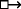. An Ł-formula is a counterfactual if its main connective is.

### Proposition 1

Let $${\mathscr{M}} = \langle P,i \rangle$$ be an S5-model (where $$i(p) \mathrel {\subseteq } P$$ for all p ∈At) and let $${\Phi }:{P}\rightarrow \wp ({\mathscr{L}})$$ map every wP to a set Φw of counterfactuals. Then there is an impossible worlds model $$\mathcal {I} = \langle {W,P,f,V}\rangle$$ such that for any wP:

1. 1.

if ϕ is an S5-formula, then $$\mathcal {I},w \Vdash _{\textsf {i}} \phi$$ iff $${\mathscr{M}},w \Vdash _{\textbf {S5}} \phi$$

2. 2.

if ψ is a counterfactual, then $$\mathcal {I},w \Vdash _{\textsf {i}} \psi$$ iff ψ ∈Φw.

### Proof

WLOG, we may assume that P is disjoint from Ł and from (Ł × P). Define $$\mathcal {I} = \langle P \cup \L \cup (\L \times P),P,f,V \rangle$$, where:

• for each p ∈At and wP, V (p,w) = 1 iff wi(p)

• for each ϕ ∈Ł and α ∈Ł, V (ϕ,α) = 1 iff α = ϕ

• for each ϕ ∈Ł and 〈α,w〉∈ (Ł × P), V (ϕ,〈α,w〉) = 1 iff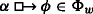• f is any selection function with the following property: if X ∩Ł = {α} and wP, then f(X,w) = {〈α,w〉}.

It is easy to establish (i) by induction. As for (ii), note that $$\llbracket {\alpha }\rrbracket ^{\mathcal {I}} \cap \L = \{\alpha \}$$, so $$f(\llbracket {\alpha }\rrbracket ^{\mathcal {I}},w) = \{\langle {\alpha ,w}\rangle \}$$. Hence,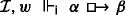iff $$\mathcal {I},\langle {\alpha ,w}\rangle \Vdash _{\textsf {i}} \beta$$, i.e., V (β,〈α,w〉) = 1, which holds iff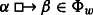. □

### Corollary 2

Let 𝜃 be any consistent S5-formula, and let 𝜃 be the result of simultaneously uniformly substituting one or more atomic sentences in 𝜃 for distinct counterfactuals. Then 𝜃 is i-satisfiable.

### Proof

Let $${q}_1, \dots , {q}_n$$ be the atomics in 𝜃 that are substituted for distinct counterfactuals $${\psi }_1, \dots , {\psi }_n$$ resulting in 𝜃. Since 𝜃 is consistent, it is S5-satisfiable. Let $${\mathscr{M}},w \Vdash _{\textbf {S5}} \theta$$. For each $$v \in W^{{\mathscr{M}}}$$, define:

$$\begin{array}{@{}rcl@{}} {\Phi}_v \mathrel{:=} \{\psi_{i} | \mathcal{M},v \Vdash_\textbf{S5} q_i\} \end{array}$$

By Proposition 1, this guarantees us an S5-equivalent impossible worlds model $$\mathcal {I}$$ such that $$\mathcal {I},v \Vdash _{\textsf {i}} \psi$$ iff ψ ∈Φv where ψ is a counterfactual. Moreover, in this model,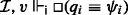. And since $$\mathcal {I},w \Vdash _{\textsf {i}} \theta$$, it follows that $$\mathcal {I},w \Vdash _{\textsf {i}} \theta ^{*}$$. □

Corollary 2 effectively says that there are no non-trivial valid inferences governing counterfactuals in the impossible worlds semantics: any inference with counterfactuals that’s i-valid is already S5-valid.

Theorem 1(b) is harder to establish. The main issue is that while hyperconventions are allowed to redefine the semantic value of the boolean connectives, they cannot touch the semantics of. But in the impossible worlds semantics, any set of Ł-formulas is satisfied at some (perhaps impossible) world in some model, including those containing counterfactuals. Thus, if we are to establish Theorem 1(b), we need to establish the expressivist analogue of Proposition 1. Indeed, this can be done, though the proof is more involved.

### Proposition 2

Let $${\mathscr{M}} = \langle W,i \rangle$$ be an S5-model and let $${\Phi }:{P}\rightarrow \wp ({\mathscr{L}})$$ map every wW to a set Φw of counterfactuals. Then there is a expressivist model $$\mathcal {E} = \langle W,f \rangle$$ and a classical hyperconvention c such that for any wW:

1. (i)

if ϕ is an S5-formula, then $$\mathcal {E},w,c \Vdash _{\exp } \phi$$ iff $${\mathscr{M}},w \Vdash _{\textbf {S5}} \phi$$

2. (ii)

if ψ is a counterfactual, then $$\mathcal {E},w,c \Vdash _{\exp } \psi$$ iff ψ ∈Φw.

### Proof

Since S5 is invariant under bisimulation contraction (and so, invariant under duplication of worlds), we may assume WLOG that W is infinite. We define c simply as the classical hyperconvention over W where c(p) = i(p) for all p ∈At.

We now set out to define f. Fix an arbitrary w0W. Let $${h}:{{\mathscr{L}}}\rightarrow {W - \{w_{0},w\}}$$ be a bijection. We’ll write wϕ in place of h(ϕ) throughout. Now, let $${\Gamma } \subseteq \L$$. Define the hyperconvention cΓ as follows (where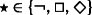and $$\circ \in \{\wedge ,\vee ,\rightarrow \}$$):

$$\begin{array}{@{}rcl@{}} c_{\Gamma}(p) & =& { \begin{cases} \{w_p,w_0\} & \text{if } p \in {\Gamma} \\ \{w_p\} & \text{otherwise} \end{cases}} \\ c_{\Gamma}(\star)(X) & =& { \begin{cases} \{w_{\star\phi} ~|~ w_\phi \in X\} \cup \{w_0\} & \parbox[t]{.5\textwidth}{if \star\phi \in {\Gamma} whenever w_{\phi} \in X} \\ \{w_{\star\phi} ~|~w_\phi \in X\} & \text{otherwise} \end{cases}} \\ c_{\Gamma}(\mathrel{\circ})(X,Y) & {=}& { {{\begin{cases} \{w_{\phi \mathrel{\circ} \psi} ~|~ w_\phi \in X \text{ and } w_\psi \in Y\} \cup \{w_0\} & \parbox[t]{.35\textwidth}{if \phi \mathrel{\circ} \psi \in {\Gamma} whenever w_{\phi} \in X and w_{\psi} \in Y} \\ \{w_{\phi \mathrel{\circ} \psi} ~|~w_\phi \in X \text{ and } w_\psi \in Y\} & \text{otherwise} \end{cases}}}} \end{array}$$

Let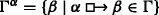. Define f as follows: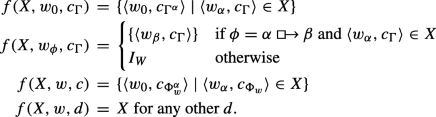Let $$\mathcal {E} = \langle W,f \rangle$$. It is easy to check that (i) holds by induction. So we just need to establish (ii). First, some intermediate claims:

### Claim 1

For any Γ and any ϕ,ψ: $$\mathcal {E},w_{\phi },c_{\Gamma } \Vdash \psi$$ iff ϕ = ψ.

### Proof

By induction. The atomic case holds by definition of cΓ. The cases for the connectives is straightforward. For the counterfactual,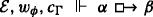iff $$f(\llbracket {\alpha }\rrbracket ^{\mathcal {E}},w_{\phi },c_{{\Gamma }}) \subseteq \llbracket {\beta }\rrbracket ^{\mathcal {E}}$$. By induction hypothesis, $$\langle {w_{\gamma },c_{{\Gamma }}}\rangle \in \llbracket {\beta }\rrbracket ^{\mathcal {E}}$$ iff γ = β. Hence, $$\llbracket {\beta }\rrbracket ^{\mathcal {E}} \neq I_{W}$$, which means $$f(\llbracket {\alpha }\rrbracket ^{\mathcal {E}},w_{\phi },c_{{\Gamma }}) \subseteq \llbracket {\beta }\rrbracket ^{\mathcal {E}}$$ iff $$f(\llbracket {\alpha }\rrbracket ^{\mathcal {E}},w_{\phi },c_{{\Gamma }}) = \{\langle {w_{\beta },c_{{\Gamma }}}\rangle \}$$, which holds iff. But again by induction hypothesis, $$\langle {w_{\alpha },c_{{\Gamma }}}\rangle \in \llbracket {\alpha }\rrbracket ^{\mathcal {E}}$$. Thus,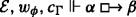iff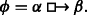. □

### Claim 2

For any Γ and any ϕ: $$\mathcal {E},w_{0},c_{\Gamma } \Vdash \phi$$ iff ϕ ∈Γ.

### Proof

By induction. The atomic case holds by definition of cΓ. The cases for the connectives is straightforward using Claim 1 and the inductive hypothesis. For the counterfactual,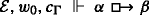iff $$f(\llbracket {\alpha }\rrbracket ^{\mathcal {E}},w_{0},c_{{\Gamma }}) \subseteq \llbracket {\beta }\rrbracket ^{\mathcal {E}}$$. By Claim 1, $$\langle {w_{\gamma },c_{{\Gamma }}}\rangle \in \llbracket {\alpha }\rrbracket ^{\mathcal {E}}$$ iff γ = α. So $$f(\llbracket {\alpha }\rrbracket ^{\mathcal {E}},w_{0},c_{{\Gamma }}) = \{\langle {w_{0},c_{{\Gamma }^{\alpha }}}\rangle \}$$. Hence, $$\mathcal {E},w_{0},c_{{\Gamma }} \Vdash$$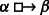iff $$\mathcal {E},w_{0},c_{{\Gamma }^{\alpha }} \Vdash \beta$$. But again by induction hypothesis, this holds iff β ∈Γα, i.e.,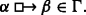. □

We are now ready to prove (ii).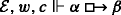iff $$f(\llbracket {\alpha }\rrbracket ^{\mathcal {E}},w,c) \subseteq \llbracket {\beta }\rrbracket ^{\mathcal {E}}$$. By Claim 1, $$\langle w_{\gamma },c_{{\Phi }_{w}} \rangle \in \llbracket {\alpha }\rrbracket ^{\mathcal {E}}$$ iff γ = α. Hence, $$f(\llbracket {\alpha }\rrbracket ^{\mathcal {E}},w,c) = \{\langle {w_{0},c_{{\Phi }_{w}^{\alpha }}}\rangle \}$$. Soiff $$\mathcal {E},w_{0},c_{{\Phi }_{w}^{\alpha }} \Vdash \beta$$, which by Claim 2 holds iff $$\beta \in {\Phi }_{w}^{\alpha }$$, i.e.,. □

### Corollary 3

Let 𝜃 be any consistent S5-formula, and let 𝜃 be the result of uniformly substituting one or more atomic sentences in 𝜃 for distinct counterfactuals. Then 𝜃 is $$\exp$$-satisfiable.

Now we can establish Theorem 1(b):

### Proof Proof (Theorem 1(b))

Let $$\mathcal {I} = \langle W,P,f,V \rangle$$ and first let wP. Let:

$$\begin{array}{@{}rcl@{}} {\Phi} & {=}& \{\phi ~|~ \phi \text{ is an \textbf{S5}-formula and } \mathcal{I},w \Vdash_\textsf{i} \phi] \\ {\Psi} & {=}& \{\phi~|~\phi \text{ is a counterfactual and } \mathcal{I},w \Vdash_\textsf{i} \phi]. \end{array}$$

By Proposition 2, there is a expressivist model $$\mathcal {I}^{\exp } = \langle W,f^{\exp } \rangle$$ and a classical hyperconvention c such that $$\mathcal {I}^{\exp },w,c \Vdash _{\exp } {\Phi } \cup {\Psi }$$ and if ϕ is a counterfactual not in Ψ, $$\mathcal {I}^{\exp },w,c \nVdash _{\exp } \phi$$. Hence, by a simple induction, $$\mathcal {I},w \Vdash _{\textsf {i}} \phi$$ iff $$\mathcal {I}^{\exp },w,c \Vdash _{\exp } \phi$$.

Now let wP. Let Γ = {ϕ | V (ϕ,w) = 1} and let $$\mathcal {I}^{\exp }$$ be 〈W,f〉 where f is constructed as in Proposition 2. Then by Claim 2, $$\mathcal {I}^{\exp },w_{0},c_{{\Gamma }} \Vdash _{\exp } \phi$$ iff ϕ ∈Γ. Hence, we can take x = 〈w0,cΓ〉. □

## Rights and permissions

Reprints and Permissions